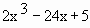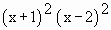Home MonkeyNotes Printable Notes Digital Library Study Guides Study Smart Parents Tips College Planning Test Prep Fun Zone Help / FAQ How to Cite New Title Request

Technique

(1) Find f '(x) and f " (x).

(2) Find the roots (i.e. critical points) of f ' (x) = 0.

Let x = a, x = b, x = c etc be these critical points of f (x).

(3) If f " (a) < 0, then f (x) has a local maximum at x = a and if f " (a) > 0, then f (x) has a local minimum at x = a. But if f " (a) = 0. Then the test fails.

(4) Use the same procedure for all critical points of f (x) as it has been given in (3).

Drawbacks :

(I)       1) f ' (x) = 0 and f" (x) = 0

2) f ' (x) = 0 and f "(x) DNE

3) f ' (x) DNE

Under any of these conditions, the first derivative test would have to be used instead the second derivative test.

(II) In case of some functions, the second derivatives are very difficult and tedious to calculate. Thus relent back to the first derivative test to find the Local extrema.

Example 20
Find the relative maxima and minima of f (x) =Solution : f (x) =\ f ' (x) = 6x2 - 24 and f " (x) = 12 x

\ Critical points are, f ' (x) = 0 Þ or

i.e. x = 2 and x = - 2

Since f " (- 2) = 12 (- 2) = - 24 < 0.

' f ' has relative maxima at x = - 2.

Thus f ( - 2) = 2 (-2)3 - 24 (-2) + 5 = 37

The relative maximum value of f (x).

Also, f " (2) = 12 (2) = 24 > 0.

Therefore 'f ' has a relative minima at x = 2 and

f (2) = 2 (2) 3 - 24 (2) + 5 = -27 the relative minimum value of f(x).Your browser does not support the IFRAME tag.

Example 21

Examine f (x) =for the relative maximum and minimum values.

Solution :Index

5.1 Tangent And Normal Lines
5.2 Angle Between Two Curves
5.3 Interpretation Of The Sign Of The Derivative
5.4 Locality Increasing Or Decreasing Functions 5.5 Critical Points
5.6 Turning Points
5.7 Extreme Value Theorem
5.8 The Mean-value Theorem
5.9 First Derivative Test For Local Extrema
5.10 Second Derivative Test For Local Extrema
5.11 Stationary Points
5.12 Concavity And Points Of Inflection
5.13 Rate Measure (distance, Velocity And Acceleration)
5.14 Related Rates
5.15 Differentials : Errors And Approximation